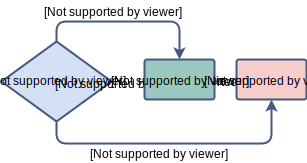a shot of dev knowledge

Related tags

# What is a ternary operator in Java?

The ternary operator is a part of Java’s conditional statements. As the name ternary suggests, it is the only operator in Java consisting of three operands.

The ternary operator can be thought of as a simplified version of the if-else statement with a value to be returned.### Syntax

The three operands in a ternary operator include:

• A boolean expression that evaluates to either true or false.
• A value to be assigned if the expression is evaluated to true.
• A value to be assigned if the expression is evaluated to false.
variable var = (booleanExpression) ? value1 if true : value2 if false

The variable var on the left-hand side of the = (assignment) operator will be assigned:

• value1 if the booleanExpression evaluates to true
• value2 if the booleanExpression evaluates to false

## Example

Let’s write a Java program to check if an integer is even or odd.

### Using if-else

class CheckEvenNumber {
public static void main( String args[] ) {
int number = 3;
if(number % 2 == 0)
{
System.out.println("The number is even!");
}
else
{
System.out.println("The number is odd!");
}
}
}

This was easy! Right? So, let’s achieve the same functionality using the Ternary operator.

### Using ternary operator

class CheckEvenNumber {
public static void main( String args[] ) {
int number = 3;
String msg =  (number % 2 == 0) ? "The number is even!" : "The number is odd!";
System.out.println(msg);
}
}

Note: Every code using if-else statement cannot be replaced with the ternary operator.

## Explanation

The above code is using the ternary operator to find if a number is even or odd.

• msg will be assigned “The number is even!” if the condition (number % 2 == 0) is true.
• msg will be assigned “The number is odd!” if the condition (number % 2 == 0) is false.

Related tags

RELATED COURSES
Related Courses
Related Courses

#### Keep Exploring

Learn in-demand tech skills in half the time

Copyright ©2021 Educative, Inc. All rights reserved.